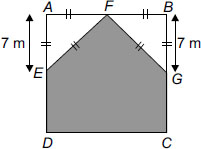# User Forum

Subject :IMO    Class : Class 5

ABCD is a square of perimeter 56 m. Two triangular corners have been cut away as shown in figure. What is the perimeter of the remaining figure?A 39 m
B 42 m
C 35 m
D 53 m

Answer is 47.8. All given answers are wrong. Triangles AEF and FBG are right angled triangles hence EF and FG need to be calculated using Pythagoras.

Class : Class 6

## Ans 2:

Class : Class 5
jow do you calculate the side of an isosceles triangle?

## Ans 3:

Class : Class 6
Ans is B because the side of the square is 56 m/4=14m. half of the two sides on the left side and the right side is being cut and the upper side is being cut totally and the two triangles are equivalent triangles. So the perimeter of the remaining part will be (7 m X 4)+14 m=28 m+14 m=42 m.

Class : Class 5